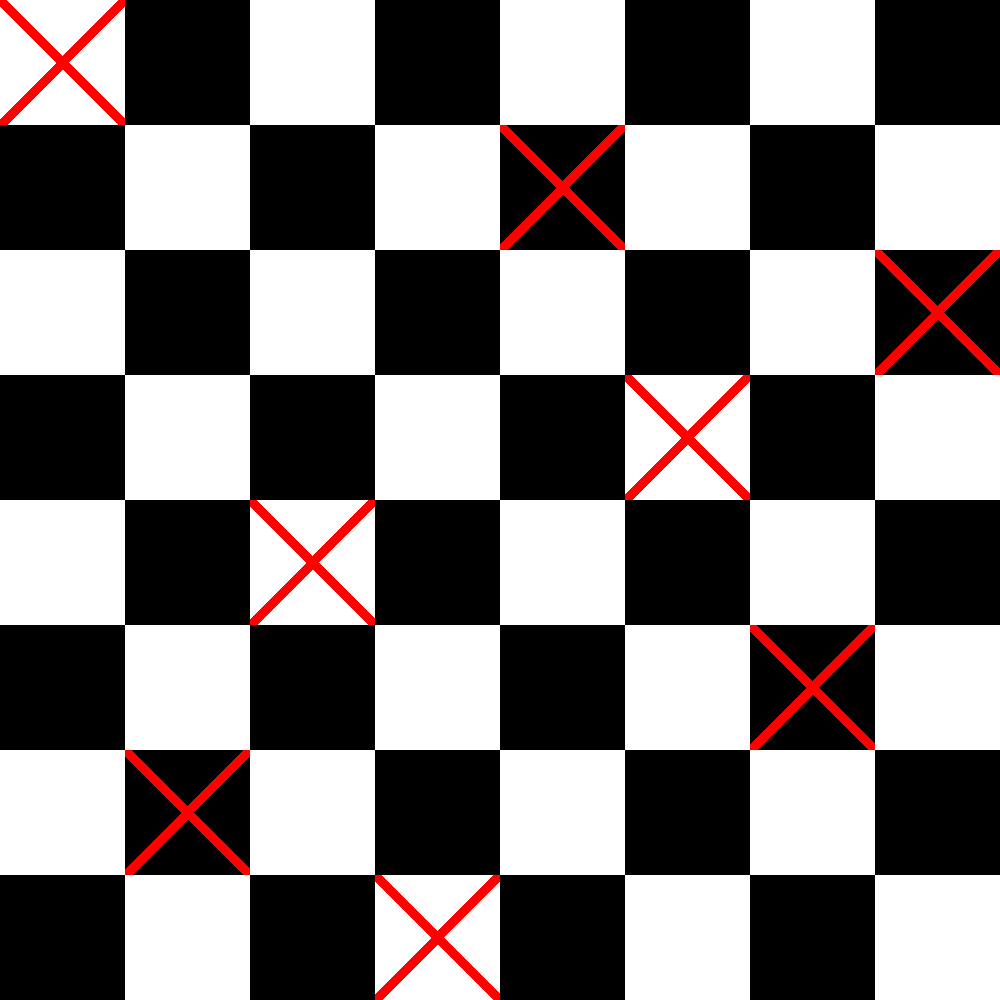· Tutorial ·

# What is the Backtracking algorithm and how to apply it in C++?

The backtracking algorithm is a powerful technique for solving combinatorial and exhaustive search problems. It is used to explore all possible solutions to a problem in a systematic way, discarding those that do not satisfy certain conditions. In this manual, we will provide you with a basic understanding of what the backtracking algorithm is and how to apply it in C++ to solve complex problems.

## 1. Backtracking Basics

Backtracking is a recursive approach that explores all possible options to find a solution to a problem. It works by breaking the problem into smaller subproblems, performing tests and backtracking when an invalid solution is found. It is especially useful for problems where it is necessary to try many different combinations to find an optimal solution.

## 2. Fundamental Steps of Backtracking

The backtracking process consists of several key steps:

1. Choice: A decision is made at the current search level, which determines how the search tree will be branched.

2. Exploration: The available options are recursively explored from the choice made.

3. Validation: Checks whether the current choice leads to a valid solution. If not, a backtrack is performed.

4. Backtrack: If the current choice does not lead to a valid solution, it is reverted to a previous state and a new choice is made.

## 3. Application in C++

To apply the backtracking algorithm in C++, we will use the Solver, Solution and Candidates classes.

### Solver class

The Solver class contains the core logic of the backtracking algorithm. It coordinates the exploration of all possible solutions, performs validation and backtracking when necessary.

### Solution class

The Solution class is responsible for storing the current state of the solution at each step of the backtracking process. This includes decisions made so far and relevant information defining the solution in progress.

### Candidates class

The Candidates class is used to generate the choices available at each step of the search. It provides an interface to obtain the possible choices based on the current state of the solution.

## Example: The N Queens Problem

A classic example of backtracking application is the N Queens problem, where N queens must be placed on a chessboard such that they do not attack each other in any direction. Here is the complete code of what the implementation would look like in C++ using the solver, solution and candidate classes:

``````#include <iostream>
#include <vector>

using namespace std;

class Solucion {
public:
Solucion(int N) : tablero(N, vector<int>(N, 0)), N(N) {}

void imprimir() const {
for (int i = 0; i < N; ++i) {
for (int j = 0; j < N; ++j) {
cout << (tablero[i][j] ? "Q" : ".");
}
cout << endl;
}
}

vector<vector<int>> tablero;
int N;
};

class Candidatos {
public:
Candidatos(int N) : disponibles(N, true) {}

vector<int> obtener() const {
vector<int> candidatos;
for (int i = 0; i < disponibles.size(); ++i) {
if (disponibles[i]) {
candidatos.push_back(i);
}
}
return candidatos;
}

void marcar(int pos) {
disponibles[pos] = false;
}

void desmarcar(int pos) {
disponibles[pos] = true;
}

private:
vector<bool> disponibles;
};

public:

bool resolverNReinas(Solucion& solucion, int fila) {
if (fila == N) {
return true;  // Todas las reinas están colocadas
}

Candidatos candidatos(N);
vector<int> cands = candidatos.obtener();

for (int columna : cands) {
if (esSeguro(solucion, fila, columna)) {
solucion.tablero[fila][columna] = 1;
candidatos.marcar(columna);

if (resolverNReinas(solucion, fila + 1)) {
return true;
}

candidatos.desmarcar(columna);
solucion.tablero[fila][columna] = 0;  // Retroceder si no se encuentra solución
}
}

return false;  // No se encontró una posición segura para colocar la reina en esta fila
}

private:
bool esSeguro(const Solucion& solucion, int fila, int columna) const {
for (int i = 0; i < fila; ++i) {
if (solucion.tablero[i][columna] == 1) {
return false;
}
}

for (int i = fila, j = columna; i >= 0 && j >= 0; --i, --j) {
if (solucion.tablero[i][j] == 1) {
return false;
}
}

for (int i = fila, j = columna; i >= 0 && j < N; --i, ++j) {
if (solucion.tablero[i][j] == 1) {
return false;
}
}

return true;
}

int N;
};

int main() {
int N;
cout << "Ingrese el tamaño del tablero (N): ";
cin >> N;

Solucion solucion(N);

cout << "Solución encontrada:" << endl;
solucion.imprimir();
} else {
cout << "No se encontró solución para N = " << N << endl;
}

return 0;
}
``````

If we run the program indicating that the board is 8X8 , we should see that the queens have been placed as follows:i